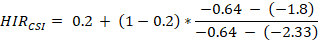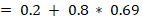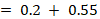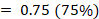﻿ Appendix 4: HIR

# Appendix 4: HIR

Hydromorphological Impact Ratios

Hydromorphological Impact Ratios (HIR) are indices describing departure from natural condition and impact for four hydromorphological indices (CSI, FRI, GAI and CVI) using the following equation: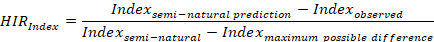Semi-natural index values were predicted using map-derived data on stream power, shear stress, geology, etc for a subset of semi-natural RHS sites (Naura et al, ms).

The HIR is a ratio between observed over maximum possible departure from natural state/impact. It varies from 0 (no impact/semi-natural condition) to 1 (maximum impact). For example, following a survey, we find that a site is dominated by sand and gravel with a CSI value of -1.8 (see figure below). Using predicted data taken from a map (see Extracting map data from nearby assessment points), we find that the semi-natural CSI should be -0.64 indicating a gravel-dominated stream. The HIRCSI will be calculated as: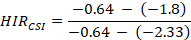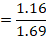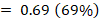Channel Substrate Index (CSI) and HIRCSI calculation. The chart represents the substrate composition of RHS sites with increasing CSI values (x-axis). The HIRCSI is calculated as the ratio between ‘observed’ and ‘maximum possible’ departure from semi-natural state or impact.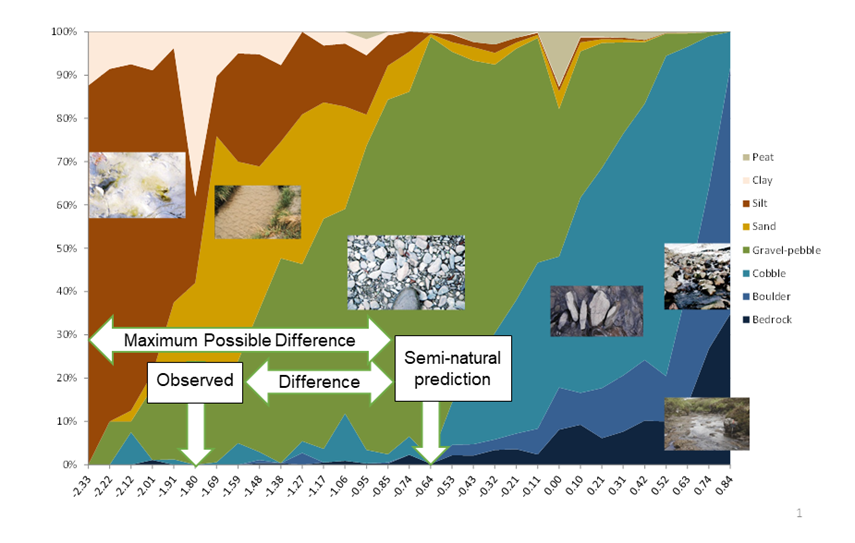The calculated HIRCSI value (0.69) means that the observed difference of 1.16 between semi-natural and observed CSI values represents 69% of the maximum possible difference (1.69) for a silt-dominated stream. The site can be considered as heavily impacted.

HIRindex values are calculated for FRI (HRIFRI), GAI (HRIGAI) and CVI ( HRICVI). The indices are categorised into five categories representing:

• ‘Very Low’ (HIRindex between 0 and 12.5%),
• ‘Low’ (HIRindex between 12.5 and 25%) ,
• ‘Moderate’ (HIRindex between 25 and 37.5%) ,
• ‘High’ (HIRindex between 37.5 and 50%)  and
• ‘Very High’ impacts (HIRindex between 50 and 100%).

A composite HIR index can then be derived for each site using the highest impact value amongst all four indices.  Thus, a site with a HRICSI, HRIFRI, HRIGAI and HRICVI of 2, 3, 3 and 5 would have an overall HIR or 5 (Very High Impact).

Note for HRICSI calculation: in the case of CSI, some of the channel substrate may have been artificially introduced such as concrete, gabions or boulders from nearby rip-rap. In this case, substrate is recorded as ‘ARtificial’ in RHS.  For the calculation, of CSI, artificial substrate is given the same score as bedrock, which results in higher CSI scores and does not accurately represent the natural substrate size.

To calculate a meaningful HRICSI score, we therefore need to account for the presence of artificial substrate. To do so, we need to:

• recalculate CSI omitting any artificial substrate and we use this value to derive the distance to semi-natural condition;
• include the coverage of artificial substrate in the HIRCSI calculation so that if 80% of the site had concrete as bed material, the HIRCSI would at least be set at 80%.

We do so by applying the following formula:Therefore, if the site in the figure above has 20% of its river bed concreted up and the rest made of gravel/sand/silt, the score would be: